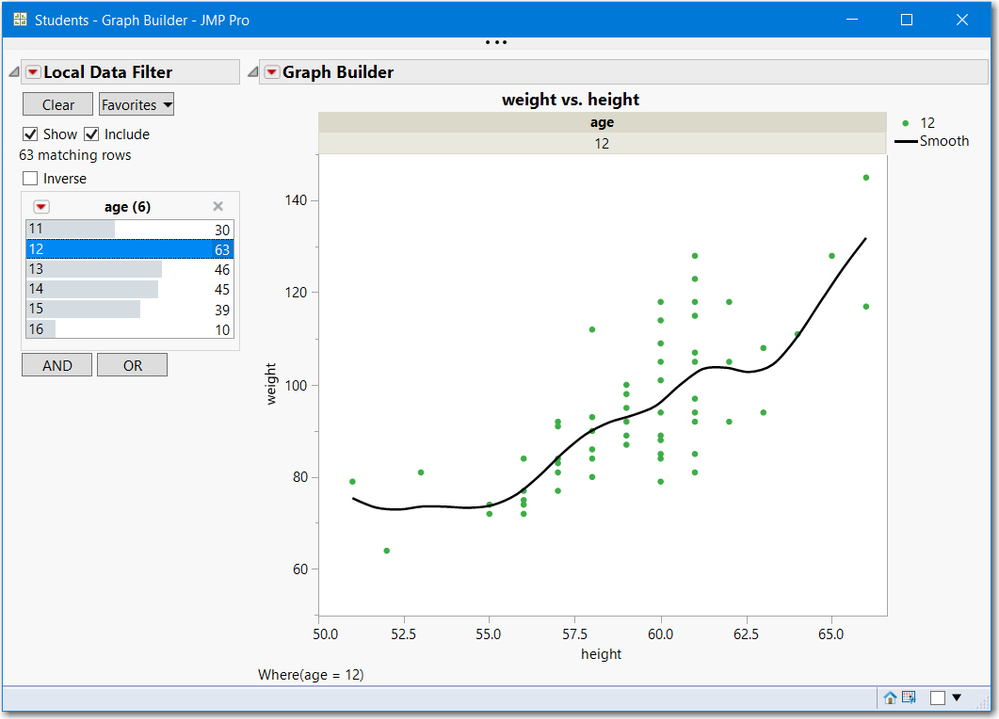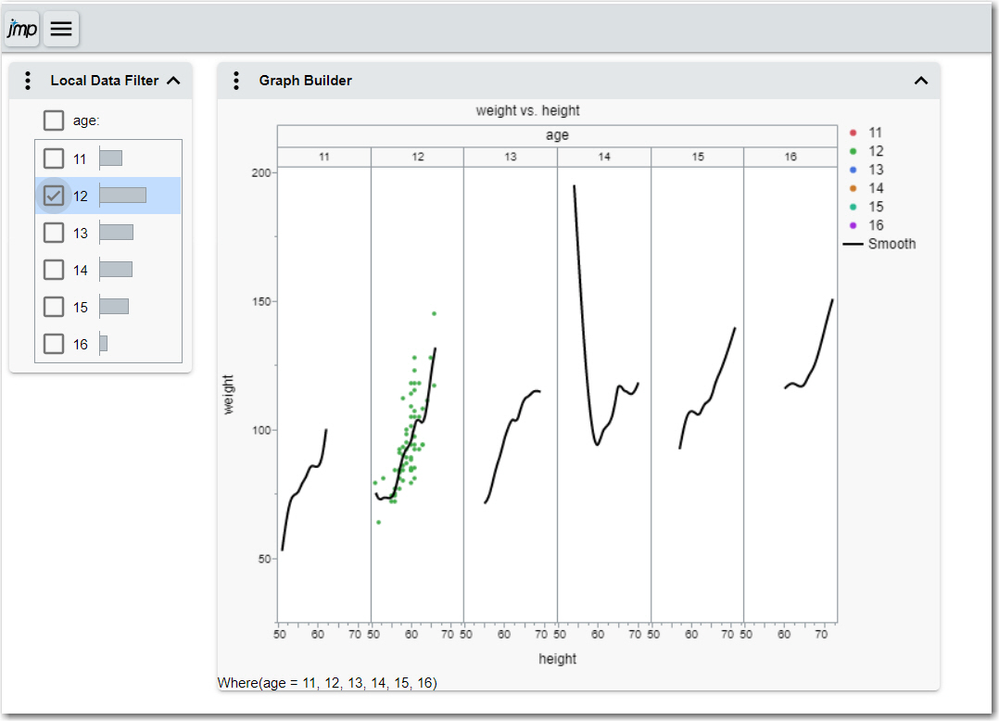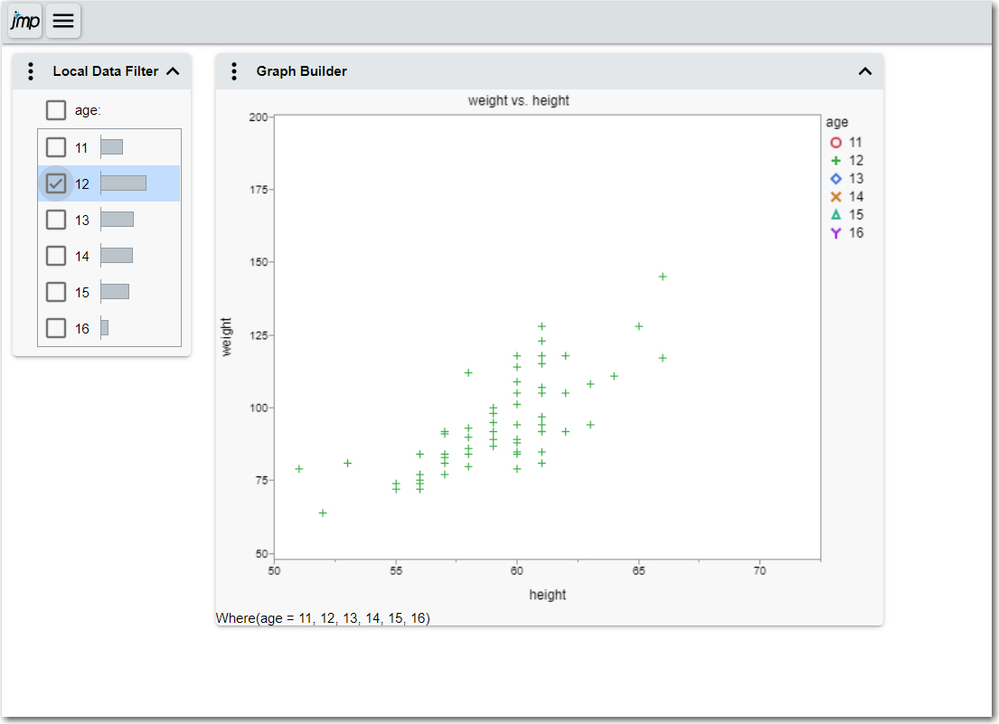Choose Language Hide Translation Bar
Highlighted

## interactive html and local data filter in jsl

Hi,

I want to save plots of the same variable but filtered for different values as interactive html.

Here is an example:

``````new_window = New Window( "NW", hlb = H List Box() );
for  (age_index=1,age_index<=N items(age_list),age_index++,
graph = Graph Builder(
invisible,
Size( 1357, 920 ),
Variables(
X( :index ),
Y( :Height ),
),
Local Data Filter(
columns( :Age ),
Where( :Age == age_list[age_index] ),)
)
);
dt << save script to data table("my_plot");
hlb << append(V List Box(dt << run script("my_plot"));
);
new_window<<save interactive html("plot");``````

In the saved html file, for the first index the plots are shown fine, for the rest of the age_indexes the axis of the plots are shown but with no data. So the plots are empty. In the open new_window when running the script, the data is shown completely. Even when I try to manually save the window as Interactive HTML, only the data of the first age_index are shown.

Is there a way to save these kinds of plots correctly in one html file in an interactive format?

3 REPLIES 3
Highlighted

## Re: interactive html and local data filter in jsl

You did not mention which version of JMP that you currently use.  In the latest version, JMP 15, Graph Builder with a Local Data Filter is only partially implemented.  For example, using the JMP Sample data table Students,  while an active window in JMP, when I select an age group, the graph points elements are dynamic. If saved as interactive HTML, however, other elements like a smoother is not.  Below is a graphbuilder plot using age as an X Group. In JMP, only the item I selected and its smoother is shown; with interactive HTML, the non-dynamic elements are fixed. More discussion below the pictures.In JMPInteractive HTML

If you only have point elements, then try this script. The key is to select all items in the list, then save the graph as interactive HTML. Then on the web you can select the points. Note the legend and note (the where statement)  are not dynamic.  See the HTML output below the script. Given that, there are multiple methods to create a web view to select a picture.

Please provide a few more details, like which JMP version you use, and whether your selection list is long, do you want all views in a horizontal list, or only one view at a time, etc.

``````Names Default to Here(1);
dt = open("\$Sample_Data/Students.jmp");

graph = dt << Graph Builder(
Size( 534, 456 ),
Show Control Panel( 0 ),
Variables( X( :height ), Y( :weight ), Overlay( :age ), Color( :age ) ),
Elements( Points( X, Y, Legend( 152 ) ) ),
Local Data Filter(
columns( :age ),
Where( :age == {11, 12, 13, 14, 15, 16} ),
Display( :age, Size( 178, 102 ), Height( 102 ) )
)
)
);
graph << save as interactive html("c:/temp/point_selection.htm");``````Highlighted

## Re: interactive html and local data filter in jsl

Thanks a lot for your suggestions and your explanations. It makes sense now.

I am using JMP 14.2.0.

I actually need the plots in one page because they should be compared with each other.

Is it possible to save each graph as interactive html, and add the saved plots to a dashboard?

My list is not long, would have around 10 elements.

Would it be possible?

Highlighted

## Re: interactive html and local data filter in jsl

For Interactive HTML you might get better results in this case by simply unchecking the "Include" checkbox allowing the data filter to simply hide/show parts of the entire dataset rather than exporting only the included portion (including and excluding rows is a feature of JMP Live).  After export you are only hiding/showing a subset of the originally included rows, not the entire dataset.  However our intention is for the initial display of the HTML output to match JMP so if that isn't the case it is a bug.  After interaction, the two might be different due to the "Include" checkbox.

In general if I am understanding your last question correctly, yes you could put several plots in one page in a dashboard.   Another user was asking about Column Switcher (which is also supported in JMP Live) and a colleague of mine proposed several workarounds for Interactive HTML such as writing a script to recreate the plot for each column and put them into a dashboard which sounds like what you want to do.  This might give you some ideas: https://community.jmp.com/t5/Discussions/How-to-add-HTML-links-to-JMP-interactive-HTML-files/m-p/232...

Article Labels

There are no labels assigned to this post.